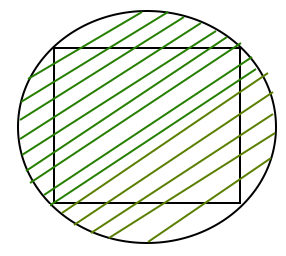Open in App
Not now

# Area of a Circumscribed Circle of a Square

• Difficulty Level : Basic
• Last Updated : 09 Jun, 2022

Given the side of a square then find the area of a Circumscribed circle around it.
Examples:

```Input : a = 6
Output : Area of a circumscribed circle is : 56.55

Input : a = 4
Output : Area of a circumscribed circle is : 25.13```

All four sides of a square are of equal length and all four angles are 90 degree. The circle is circumscribed on a given square shown by a shaded region in the below diagram.Properties of Circumscribed circle are as follows:

• The center of the circumcircle is the point where the two diagonals of a square meet.
• Circumscribed circle of a square is made through the four vertices of a square.
• The radius of a circumcircle of a square is equal to the radius of a square.

Formula used to calculate the area of inscribed circle is:
(PI * a * a)/2
where, a is the side of a square in which a circle is circumscribed.
How does this formula work?
We know area of circle = PI*r*r.
We also know radius of circle = (square diagonal)/2
Length of diagonal = ?(2*a*a)
Area = PI*r*r = (PI*a*a)/2

## C++

 `// C++ Program to find the` `// area of a circumscribed circle` `#include ` `#define PI 3.14159265`   `float` `areacircumscribed(``float` `a)` `{` `    ``return` `(a * a * (PI / 2));` `}`   `// Driver code` `int` `main()` `{` `    ``float` `a = 6;` `    ``printf``(``" Area of an circumscribed circle is : %.2f "``,` `           ``areacircumscribed(a));` `    ``return` `0;` `}`

## Java

 `// Java program to calculate` `// area of a circumscribed circle-square` `import` `java.io.*;` `class` `Gfg {` `    ``// Utility Function` `    ``static` `float` `areacircumscribed(``float` `a)` `    ``{` `        ``float` `PI = ``3``.14159265f;` `        ``return` `(a * a * (PI / ``2``));` `    ``}`   `    ``// Driver Function` `    ``public` `static` `void` `main(String arg[])` `    ``{` `        ``float` `a = ``6``;` `        ``System.out.print(``"Area of an circumscribed"` `                         ``+ ``"circle is :"``);` `        ``System.out.println(areacircumscribed(a));` `    ``}` `}`   `// The code is contributed by Anant Agarwal.`

## Python3

 `# Python3 Program to find the` `# area of a circumscribed circle` `PI ``=` `3.14159265`   `def` `areacircumscribed(a):`   `    ``return` `(a ``*` `a ``*` `(PI ``/` `2``)) `   `# Driver code` `a ``=` `6` `print``(``" Area of an circumscribed circle is :"``,` `        ``round``(areacircumscribed(a), ``2``))` `        `  `# This code is contributed by Smitha Dinesh Semwal`

## C#

 `// C# Program to find the` `// area of a circumscribed circle` `using` `System;`   `class` `GFG {` `    `  `    ``public` `static` `double` `PI= 3.14159265 ;`   `    ``static` `float` `areacircumscribed(``float` `a)` `    ``{` `        ``return` `(a * a * (``float``)(PI / 2));` `    ``}` `    `  `    ``// Driver code` `    ``public` `static` `void` `Main()` `    ``{` `        ``float` `a = 6; ` `        `  `        ``Console.Write(``" Area of an circumscribed"` `                            ``+ ``" circle is : {0}"``, ` `             ``Math.Round(areacircumscribed(a), 2));` `    ``} ` `}`   `// This code is contributed by ` `// Smitha Dinesh Semwal`

## PHP

 ``

## Javascript

 ``

Output

` Area of an circumscribed circle is : 56.55 `

Time Complexity: O(1)
Auxiliary Space: O(1)

My Personal Notes arrow_drop_up
Related Articles